# Welcome to Everyday Math Information Session about Everyday

• Slides: 16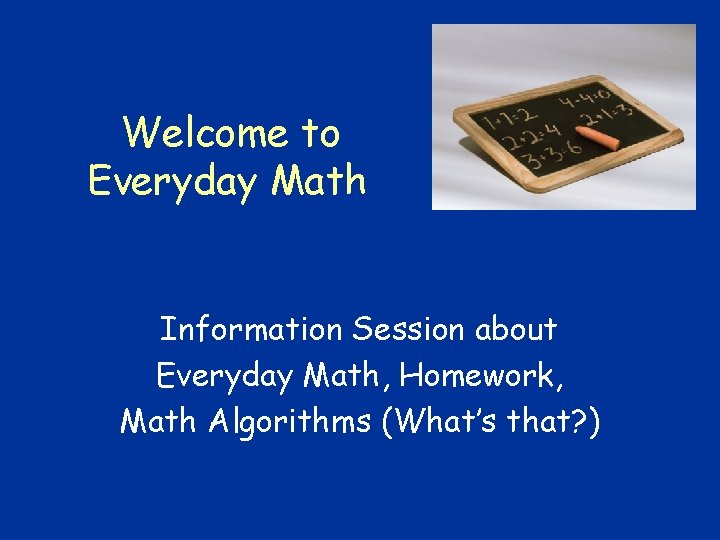Welcome to Everyday Math Information Session about Everyday Math, Homework, Math Algorithms (What’s that? )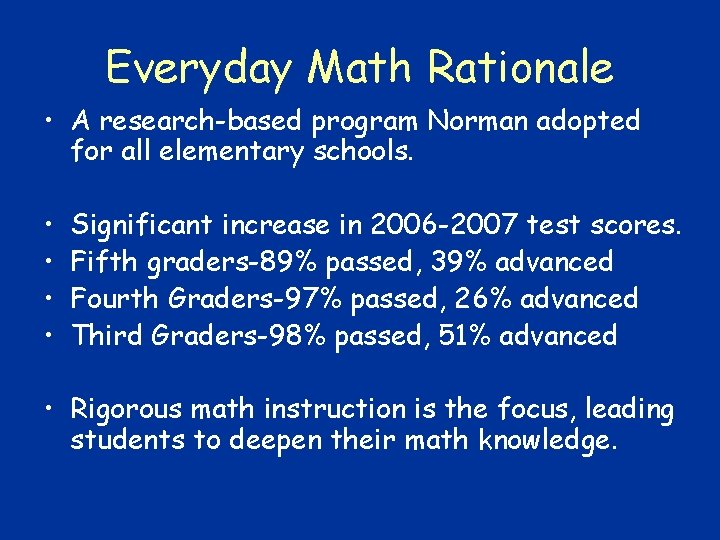Everyday Math Rationale • A research-based program Norman adopted for all elementary schools. • • Significant increase in 2006 -2007 test scores. Fifth graders-89% passed, 39% advanced Fourth Graders-97% passed, 26% advanced Third Graders-98% passed, 51% advanced • Rigorous math instruction is the focus, leading students to deepen their math knowledge.Everyday Math • Students will – discuss math strategies, – write about math activities, – practice math through challenging games, – use math materials in meaningful ways to solve problems, – understand measurement, geometry, probability, estimation, – accurately memorize & understand basic facts, – organize data, – develop money-smarts, – and reason logically.Math instruction includes a LOT more than it did when we were in school…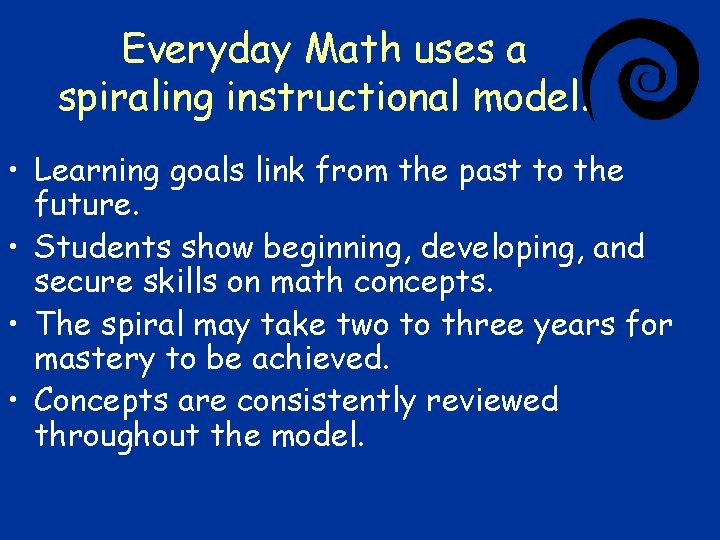Everyday Math uses a spiraling instructional model. • Learning goals link from the past to the future. • Students show beginning, developing, and secure skills on math concepts. • The spiral may take two to three years for mastery to be achieved. • Concepts are consistently reviewed throughout the model.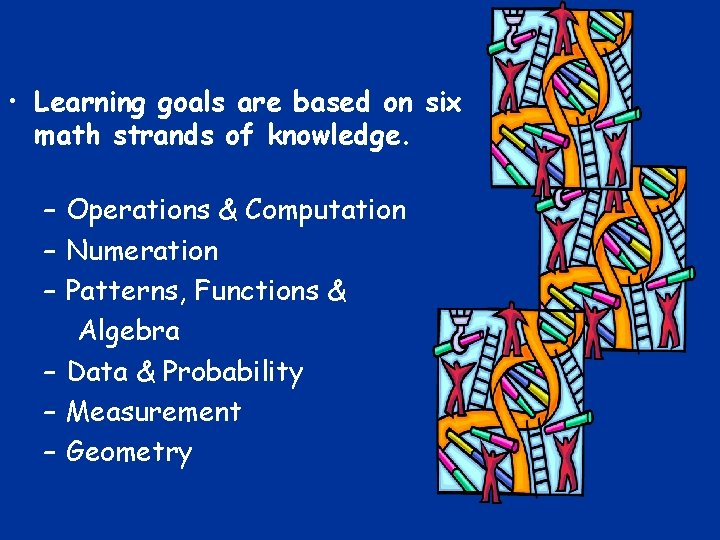• Learning goals are based on six math strands of knowledge. – Operations & Computation – Numeration – Patterns, Functions & Algebra – Data & Probability – Measurement – GeometryWhat about homework? When each unit begins, a parent information letter is sent home explaining math ideas for the unit. Shhh! (It has all homework answers for the unit. ) KEEP THIS LETTER IN A SAFE PLACE!• Your child will receive a homework assignment for each lesson in every unit. • Intermediate grade work is called “Study Links. ”More Homework Info • Everyday Math work may seem very different than math we did when we were in school. • If you and/or your child do not understand the homework, write a note to the teacher and help will be provided. • We expect and respect parent questions.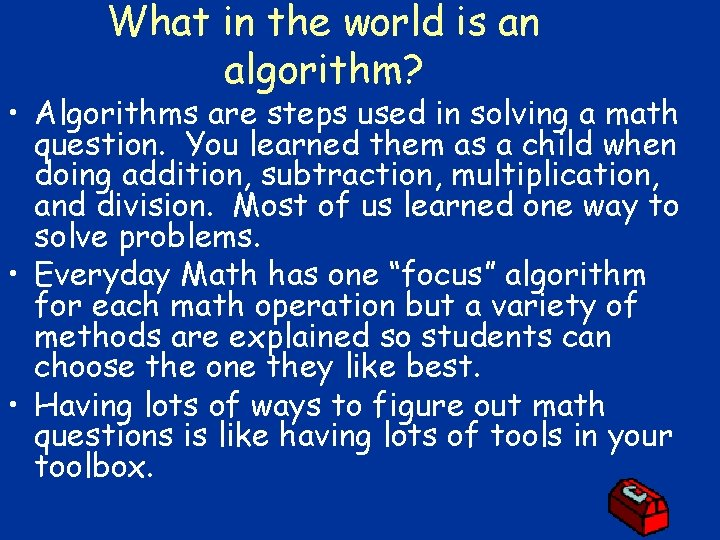What in the world is an algorithm? • Algorithms are steps used in solving a math question. You learned them as a child when doing addition, subtraction, multiplication, and division. Most of us learned one way to solve problems. • Everyday Math has one “focus” algorithm for each math operation but a variety of methods are explained so students can choose the one they like best. • Having lots of ways to figure out math questions is like having lots of tools in your toolbox.Partial Sums Addition • Add one place-value column at a time. • Write each partial sum below the problem. • Then add all partial sums to find the total. 835 + 243 (800 + 200) 1, 000 (30 + 40) 70 (5 + 3) 8 Add partial sums 1, 078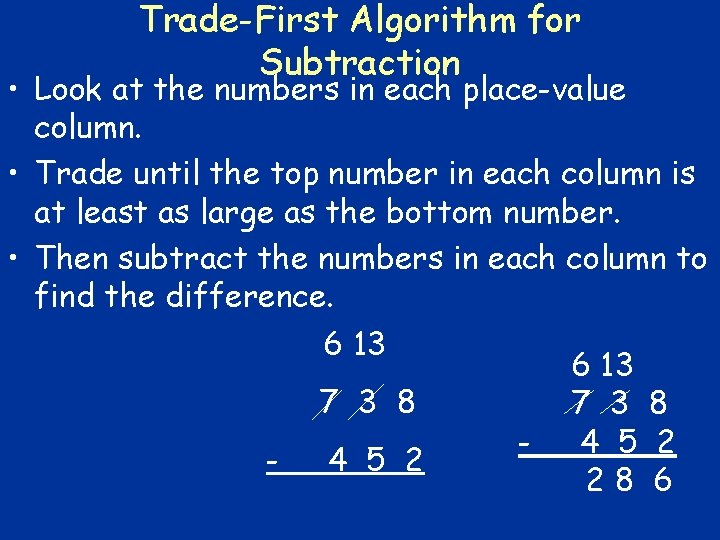Trade-First Algorithm for Subtraction • Look at the numbers in each place-value column. • Trade until the top number in each column is at least as large as the bottom number. • Then subtract the numbers in each column to find the difference. 6 13 7 3 8 - 4 5 2 28 6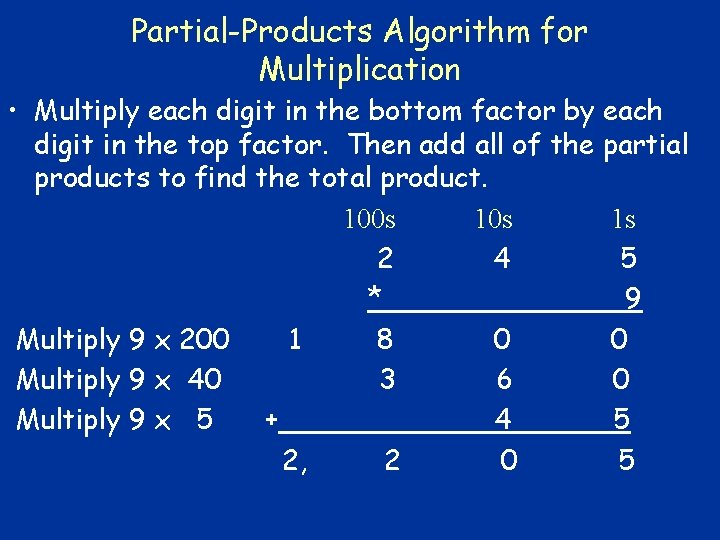Partial-Products Algorithm for Multiplication • Multiply each digit in the bottom factor by each digit in the top factor. Then add all of the partial products to find the total product. 100 s 1 s 2 4 5 * 9 Multiply 9 x 200 1 8 0 0 Multiply 9 x 40 3 6 0 Multiply 9 x 5 + 4 5 2, 2 0 5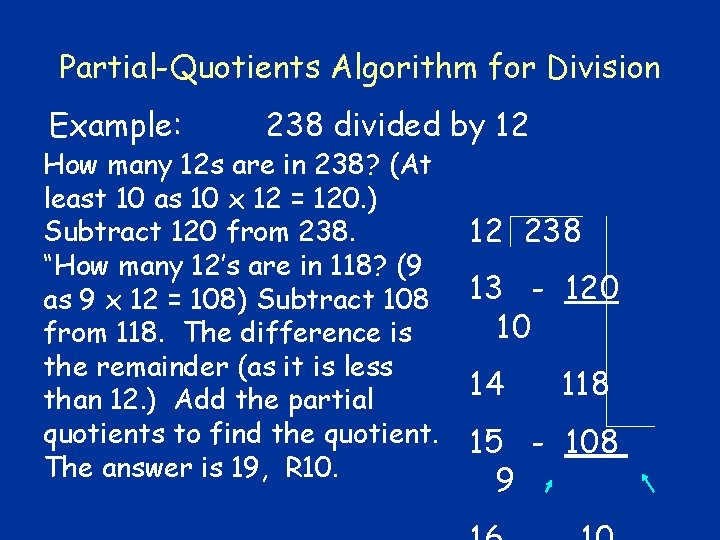Partial-Quotients Algorithm for Division Example: 238 divided by 12 How many 12 s are in 238? (At least 10 as 10 x 12 = 120. ) Subtract 120 from 238. “How many 12’s are in 118? (9 as 9 x 12 = 108) Subtract 108 from 118. The difference is the remainder (as it is less than 12. ) Add the partial quotients to find the quotient. The answer is 19, R 10. 12 238 13 - 120 10 14 118 15 - 108 9